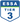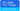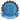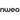# How to Solve Math Problems Faster: 15 Techniques to Show Students

All Posts“Test time. No calculators.”

You’ll intimidate many students by saying this, but teaching techniques to solve math problems with ease and speed can make it less daunting.

This can also make math more rewarding. Instead of relying on calculators, students learn strategies that can improve their concentration and estimation skills while building number sense. And, while there are educators who oppose math “tricks” for valid reasons, proponents point to benefits such as increased confidence to handle difficult problems.

Here are 15 techniques to show students, helping them solve math problems faster:Many students struggle when learning to add integers of three digits or higher together, but changing the process’s steps can make it easier.

The first step is to add what’s easy. The second step is to add the rest.

Let’s say students must find the sum of 393 and 89. They should quickly see that adding 7 onto 393 will equal 400 — an easier number to work with. To balance the equation, they can then subtract 7 from 89.

Broken down, the process is:

• 393 + 89
• (393 + 7) + (89 – 7)
• 400 + 82
• 482

With this fast technique, big numbers won’t look as scary now.

### 2. Two-Step Subtraction

There’s a similar method for subtraction.

Remove what’s easy. Then remove what’s left.

Suppose students must find the difference of 567 and 153. Most will feel that 500 is a simpler number than 567. So, they just have to take away 67 from the minuend — 567 — and the subtrahend — 153 — before solving the equation.

Here’s the process:

• 567 – 153
• (567 – 67) – (153 – 67)
• 500 – 86
• 414

Instead of two complex numbers, students will only have to tackle one.### 3. Subtracting from 1,000

You can give students confidence to handle four-digit integers with this fast technique.

To subtract a number from 1,000, subtract that number’s first two digits from 9. Then, subtract the final digit from 10.

Let’s say students must solve 1,000 – 438. Here are the steps:

• 9 – 4 = 5
• 9 – 3 = 6
• 10 – 8 = 2
• 562

This also applies to 10,000, 100,000 and other integers that follow this pattern.

## Multiplication and DivisionWhen students have to multiply two integers, they can speed up the process when one is an even number. They just need to halve the even number and double the other number.

Students can stop the process when they can no longer halve the even integer, or when the equation becomes manageable.

Using 33 x 48 as an example, here’s the process:

• 66 x 24
• 132 x 12
• 264 x 6
• 528 x 3
• 1,584

The only prerequisite is understanding the 2 times table.

### 5. Multiplying by Powers of 2

This tactic is a speedy variation of doubling and halving.

It simplifies multiplication if a number in the equation is a power of 2, meaning it works for 2, 4, 8, 16 and so on.

Here’s what to do: For each power of 2 that makes up that number, double the other number.

For example, 9 x 16 is the same thing as 9 x (2 x 2 x 2 x 2) or 9 x 24. Students can therefore double 9 four times to reach the answer:

• 9 x 24
• 18 x 23
• 36 x 22
• 72 x 2
• 144

Unlike doubling and halving, this technique demands an understanding of exponents along with a strong command of the 2 times table.### 6. Multiplying by 9

For most students, multiplying by 9 — or 99, 999 and any number that follows this pattern — is difficult compared with multiplying by a power of 10.

But there’s an easy tactic to solve this issue, and it has two parts.

First, students round up the 9  to 10. Second, after solving the new equation, they subtract the number they just multiplied by 10 from the answer.

For example, 67 x 9 will lead to the same answer as 67 x 10 – 67. Following the order of operations will give a result of 603. Similarly, 67 x 99 is the same as 67 x 100 – 67.

Despite more steps, altering the equation this way is usually faster.There’s an easier way for multiplying two-digit integers by 11.

Let’s say students must find the product of 11 x 34.

The idea is to put a space between the digits, making it 3_4. Then, add the two digits together and put the sum in the space.

What happens if the sum is two digits? Students would put the second digit in the space and add 1 to the digit to the left of the space. For example:

• 11 x 77
• 7_(7+7)_7
• 7_(14)_7
• (7+1)_4_7
• 847

It’s multiplication without having to multiply.

### 8. Multiplying Even Numbers by 5

This technique only requires basic division skills.

There are two steps, and 5 x 6 serves as an example. First, divide the number being multiplied by 5 — which is 6 — in half. Second, add 0 to the right of number.

The result is 30, which is the correct answer.

It’s an ideal, easy technique for students mastering the 5 times table.

### 9. Multiplying Odd Numbers by 5

This is another time-saving tactic that works well when teaching students the 5 times table.

This one has three steps, which 5 x 7 exemplifies.

First, subtract 1 from the number being multiplied by 5, making it an even number. Second, cut that number in half — from 6 to 3 in this instance. Third, add 5 to the right of the number.

Who needs a calculator?

### 10. Squaring a Two-Digit Number that Ends with 1Squaring a high two-digit number can be tedious, but there’s a shortcut if 1 is the second digit.

There are four steps to this shortcut, which 812 exemplifies:

• Subtract 1 from the integer: 81 – 1 = 80
• Square the integer, which is now an easier number: 80 x 80 = 6,400
• Add the integer with the resulting square twice: 6,400 + 80 + 80 = 6,560
• Add 1: 6,560 + 1 = 6,561

This work-around eliminates the difficulty surrounding the second digit, allowing students to work with multiples of 10.

### 11. Squaring a Two-Digit Numbers that Ends with 5

Squaring numbers ending in 5 is easier, as there are only two parts of the process.

First, students will always make 25 the product’s last digits.

Second, to determine the product’s first digits, students must multiply the number’s first digit — 9, for example — by the integer that’s one higher — 10, in this case.

So, students would solve 952 by designating 25 as the last two digits. They would then multiply 9 x 10 to receive 90. Putting these numbers together, the result is 9,025.

Just like that, a hard problem becomes easy multiplication for many students.

### 12. Calculating Percentages

Cross-multiplication is an important skill to develop, but there’s an easier way to calculate percentages.

For example, if students want to know what 65% of 175 is, they can multiply the numbers together and move the decimal place two digits to the left.

The result is 113.75, which is indeed the correct answer.

This shortcut is a useful timesaver on tests and quizzes.

### 13. Balancing AveragesTo determine the average among a set of numbers, students can balance them instead of using a complex formula.

Suppose a student wants to volunteer for an average of 10 hours a week over a period of four weeks. In the first three weeks, the student worked for 10, 12 and 14 hours.

To determine the number of hours required in the fourth week, the student must add how much he or she surpassed or missed the target average in the other weeks:

• 14 hours – 10 hours = 4 hours
• 12 – 10 = 2
• 10 – 10 = 0
• 4 hours + 2 hours + 0 hours = 6 hours

To learn the number of hours for the final week, the student must subtract the sum from the target average:

• 10 hours – 6 hours = 4 hours

With practice, this method may not even require pencil and paper. That’s how easy it is.## Word Problems

### 14. Identifying Buzzwords

Students who struggle to translate word problems into equations will benefit from learning how to spot buzzwords — phrases that indicate specific actions.

This isn’t a trick. It’s a tactic.

Teach students to look for these buzzwords, and what skill they align with in most contexts:

Be sure to include buzzwords that typically appear in their textbooks (or other classroom math books), as well as ones you use on tests and assignments.

As a result, they should have an easier time processing word problems.

### 15. Creating Sub-QuestionsFor complex word problems, show students how to dissect the question by answering three specific sub-questions.

Each student should ask him or herself:

• What am I looking for? — Students should read the question over and over, looking for buzzwords and identifying important details.
• What information do I need? — Students should determine which facts, figures and variables they need to solve the question. For example, if they determine the question is rooted in subtraction, they need the minuend and subtrahend.
• What information do I have? — Students should be able to create the core equation using the information in the word problem, after determining which details are important.

These sub-questions help students avoid overload.

Instead of writing and analyzing each detail of the question, they’ll be able to identify key information. If you identify students who are struggling with these, you can use peer learning as needed.

For more fresh approaches to teaching math in your classroom, consider treating your students to a range of fun math activities.

## Final Thoughts About these Ways to Solve Math Problems Faster

Showing these 15 techniques to students can give them the confidence to tackle tough questions.

They’re also mental math exercises, helping them build skills related to focus, logic and critical thinking.

A rewarding class equals an engaging class. That’s an easy equation to remember.

>Create or log into your teacher account on Prodigy — a free, adaptive math game that adjusts content to accommodate player trouble spots and learning speeds. Aligned to US and Canadian curricula, it’s loved by more than 500,000 teachers and 15 million students.

••••